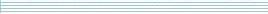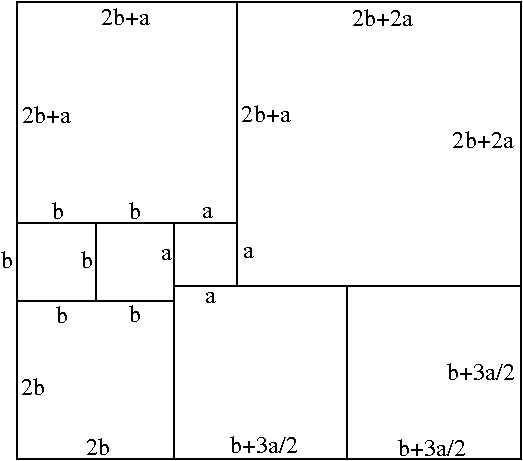| home      | people     | research     | publications    | seminars    | events     | contactCommunication Networks   | Systems Biology   | Hybrid Systems    | Machine Learning   | Dynamics & Interaction### Schools Mathematics Grand Challenge

Week six's Puzzles

Problem 16:

The solution was:

Hopefully you spotted the hint that we want to pair up the small numbers with the big ones and hope that we get something for which the sum of the digits is constant.

So you could try 1 paired up with 1,000,000, 2 paired up with 999,999, 3 paired up with 999,998 etc, but this doesn't give a constant sum of digits.

The next thing to try would be 1 paired up with 999,999, 2 paired up with 999,998, which looks good as both of these have the sum of digits being 55. However if you continue you will get to 10 paired up with 999,990, which has the sum of digits being 46, not 55, unfortunately.

Third time lucky! How about 1 paired up with 999,998, 2 paired up with 999,997, and so on? This works and all pairs add up to 6x9=54. (For example, 314159 paired up with 685840 works because (3+6) + (1+8) + (4+5) + (1+8) + (5+4) + (9+0) = 9 + 9 + 9 + 9 + 9+ 9 = 54.)

There will be 499,999 such pairs, and we mustn't forget the extra 1 from the 1,000,000 and the 54 from 999,999 which we didn't pair up, so the answer is 499,999 x 54 + 54 + 1=27,000,001

Problem 17:

The solution was:

If we label the sides of the two smallest squares with a and b and try to label as many of the other sides as possible in terms of a and b, using the fact that the fields are all square, we end up with the following picture, to scale this time!If we equate the two vertical sides of the big rectangle, we get 5b+a=3b+7a/2, which we can solve to get b=5a/4. We know that the width is 32, so 4b+3a=32. Since we know b in terms of a we can substitute and solve to get a=4, b=5, and the answer is 5b+a = 29.

Problem 18:

The solution was:

First let's figure out how much money Mary got from her mother. If you are lazy like me you might find all 21 primes between 100 and 200, square them, and get the remainder when you divide by 8. It is always one.

For the industrious, let p be the prime and let r be the remainder when you divide p by 8. The remainder when you divide p x p by 8 equals the remainder when you divide r x r by 8. But the only possible values for r are 1,3,5 or 7 (since p is prime, it must be odd). So the only possible values for r x r are 1, 9, 25, or 49. But the remainders when you divide these by 8 are always 1.

Phew. After all that, we know Mary got €10 from her mother.

Now let's think about the prices of ropes; let's start with the easy ones - 3, 13, 23, 33, 43,... 93, 103, 113,..., 193 are all free. Lazy people will immediately correctly assume Mary chose 103 or 113 or 163 or 173 or 193 as her prime and has €10 change!

Again for the industrious, denote by pr(q) the price of a rope of length q. Let p be any prime. An important rope length here is 9. What is pr(9)? pr(9)= pr(3)+pr(3) = 0. Similarly pr(27) = pr(9)+pr(3)=0. The trick here to get all primes is to think about pr(3p) and pr(9p). We know pr(3p) = pr(3) +pr(p) = pr(p), and we know pr(9p) = pr(9) +pr(p) = pr(p). But if a prime's last digit is not 3, its last digit is 1,7, or 9.

If its last digit is 1, when we multiply it by 3 its last digit will be 3, and it will be free. For example, if p=101, then 0=pr(303)= pr(3)+pr(101) = pr(101). So pr(101)=0.

If the prime's last digit is 7, we can follow 0=pr(963)=pr(9)+pr(107), so pr(107)=0.

If the prime's last digit is 9, we can follow 0=pr(2943)=pr(27)+pr(109), so pr(109)=0.

So, the cost of all prime ropes is zero and she always has €10 change.

(This question is essentially an introduction to Modular arithmetic).